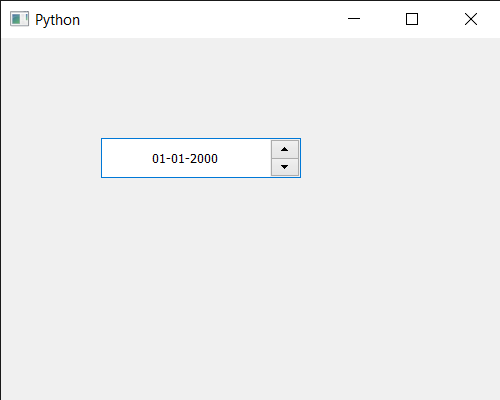Open In App

# PyQt5 QDateEdit – Setting Date Alignment

In this article we will see how we can set the date alignment of the QDateEdit. By default the text is aligned on the left-hand side although by changing the alignment we can make the date appear in the center. Changing alignment does not effect the functionality of the date edit.

In order to do this we use `setAlignment` method with the QDateEdit object

Syntax : date.setAlignment(a_flag)

Argument : It takes alignment flag as argument

Return : It returns None

Below is the implementation

 `# importing libraries``from` `PyQt5.QtWidgets ``import` `*` `from` `PyQt5 ``import` `QtCore, QtGui``from` `PyQt5.QtGui ``import` `*` `from` `PyQt5.QtCore ``import` `*``import` `sys`` ` ` ` `class` `Window(QMainWindow):`` ` `    ``def` `__init__(``self``):``        ``super``().__init__()`` ` `        ``# setting title``        ``self``.setWindowTitle(``"Python "``)`` ` `        ``# setting geometry``        ``self``.setGeometry(``100``, ``100``, ``500``, ``400``)`` ` `        ``# calling method``        ``self``.UiComponents()`` ` `        ``# showing all the widgets``        ``self``.show()`` ` `    ``# method for components``    ``def` `UiComponents(``self``):`` ` `        ``# creating a QDateEdit widget``        ``date ``=` `QDateEdit(``self``)`` ` `        ``# setting geometry of the date edit``        ``date.setGeometry(``100``, ``100``, ``200``, ``40``)`` ` `        ``# alignment``        ``a_flag ``=` `Qt.AlignCenter`` ` `        ``# setting alignment of date``        ``date.setAlignment(a_flag)`` ` `         ` ` ` ` ` ` ` `# create pyqt5 app``App ``=` `QApplication(sys.argv)`` ` `# create the instance of our Window``window ``=` `Window()`` ` `# start the app``sys.exit(App.``exec``())`

Output :